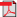# Search Results

1. P. Lassila, Efficient simulation techniques for multiservice loss systems, Licentiate thesis, Helsinki University of Technology, Networking Laboratory, 1999(bib)
Abstract: Modern broadband networks have been designed to integrate several service types into the same network. On the call scale, the process describing the number of calls present in the network can be modeled by a loss system. In principle, loss systems are mathematically simple and well understood, and one is able to write down exact expressions for such things as the blocking probability of a call belonging to a given class. However, for systems of realistic size in terms of the number and the capacity of the links and the number of traffic classes, such analytical expressions defy a direct evaluation because of the huge size of the state space. In such situations, one has to resort to simulations in order to obtain estimates of the performance measures of interest. In this thesis we are specifically dealing with identifying efficient simulation methods for estimating the blocking probabilities. To this end, we first consider some basic simulation methods and we show how they are applied for generating samples in the simulation of loss systems. In particular, from the class of static Monte Carlo methods we present a rejection sampling method for generating independent samples and the Gibbs sampler for producing dependent samples. From the class of process simulation methods, we give a number different Markov chain methods. Also, we compare each method with respect to their efficiency in terms of the variance of the estimators and the computational effort associated with the methods. Then we address the problem of increasing the efficiency of the simulations by using so called variance reduction techniques. In particular, we present two different methods for estimating the blocking probabilities in a multiservice loss system. The first method is an importance sampling method and utilizes some large deviation results for multidimensional random variables. We derive a composite sampling distribution, which is a weighted combination of distributions for effectively sampling the blocking states associated with each link in the network. We also provide heuristics to fix the weights in the composite distribution. This method is shown to be especially useful when estimating small blocking probabilities. The second method is based on another known method called conditional expectation method, where the idea is to utilize known analytical results to the maximum degree. The method is based on conditioning on the samples hitting certain one-dimensional subsets of the state space for which conditional expectations can be calculated analytically. Moreover these expectations can be precomputed and, hence, the utilization of the method does not cause much extra computational work. In effect, it eliminates the internal variance within each subset. The method is also independent of the method used for generating samples. The numerical results confirm the efficiency of the method.